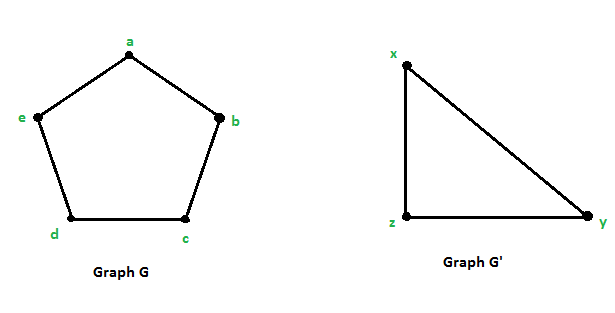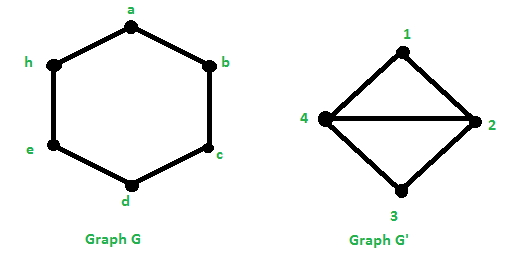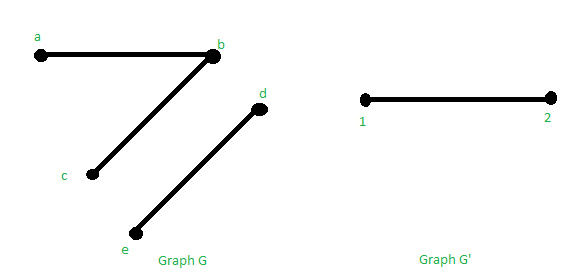Open in App
Not now

# Graph Homomorphism

• Difficulty Level : Basic
• Last Updated : 04 Jul, 2021

A graph G is a collection of a set of vertices and a set of edges that connects those vertices. It consists of two sets:

• Set of Vertices: V = {v1, v2, …, vn}
• Set of edges: E = {e1, e2, …, en}

The graph G is denoted as G = (V, E).

Homomorphism of Graphs: A graph Homomorphism is a mapping between two graphs that respects their structure, i.e., maps adjacent vertices of one graph to the adjacent vertices in the other. A homomorphism from graph G to graph H is a map from VG to VH which takes edges to edges.

Definition: A graph homomorphism F from a graph G = (V, E) to a graph G’ = (V’, E’) is written as:

f : G –> G’
It is a mapping f: V –> V’ from the vertex set of G to the vertex set of G’ such that {u, v} ∈ E ⇒ {f(u), f(v) ∈ E’

The above definition is extended to the directed graphs. Then, for a homomorphism f: G –> G’ is; {f(u), f(v)} is an arc of G’ only if (u, v) is an arc of G. If there exists a homomorphism; f: G –> G’, then it is written as G–>G’ G is said to be homomorphic to G’.

Isomorphism: If the homomorphism f: G –> G’ is a bijection (one-one and onto mapping) whose inverse is also a graph homomorphism, then f is a graph isomorphism.

Example 1: Below are the 2 graphs G = (V, E) with V = {a, b, c, d, e} and E = {(a, b), (b, c), (c, d), (d, e), (e, a)} and G’ = (V’, E’) with V’ = {x, y, z} and E’ = {(x, y), (y, z), (z, x)}.There exists a mapping f: G –> G’ such that {u, v} ∈ E ⇒ {f(u), f(v)} ∈ E’.

Solution:

Let us say that f(a) = x, f(b) = y, f(c) = z, f(d) = x and f(e) = z.

• If (a, b) is an edge in G, then (f(a), f(b)) must be an edge in E’.
`f(a) = x and f(b) = y  ⇒  (f(a), f(b)) = (x, y) ∈ E'`
• If (b, c) is an edge in G, then (f(b), f(c)) must be an edge in E’.
`f(b) = y and f(c) = z  ⇒  (f(b), f(c)) = (y, z) ∈ E'`
• If (c, d) is an edge in G, then (f(c), f(d)) must be an edge in E’.
`f(c) = z and f(d) = x  ⇒  (f(c), f(d)) = (z, x) ∈ E'`
• If (d, e) is an edge in G, then (f(d), f(e)) must be an edge in E’
`f(d) = x and f(e) = z  ⇒  (f(d), f(e)) = (x, z) ∈ E'`
• If (e, a) is an edge in G, then (f(e), f(a)) must be an edge in E’
`f(e) = z and f(a) = x  ⇒  (f(c), f(d)) = (z, x) ∈ E'`

Therefore, it can be seen that ∀{u, v} ∈ E ⇒ ∃{f(u), f(v)} ∈ E’. So f is a homomorphism.

Example 2: Below are the 2 graphs G = (V, E) with V = {a, b, c, d, e, h} and E = {(a, b), (b, c), (c, d), (d, e), (e, h), (f, a) } and G’ = (V’, E’) with V’ = {1, 2, 3, 4} and
E’ = { (1, 2), (2, 3), (3, 4), (4, 1), (4, 2)}.There exists a mapping : G–> G’ such that {u, v} ∈ E ⇒ {f(u), f(v)} ∈ E’.

Solution:

Let us say that f(a) = 1, f(b) = 4, f(c) = 2, f(d) = 4, f(e) = 2 and f(h) =  4.

• If (a, b) is an edge in G, then (f(a), f(b)) must be an edge in E’.
`f(a) = 1 and f(b) = 4  ⇒  (f(a), f(b)) = (1, 4) ∈ E'`
• If (b, c) is an edge in G, then (f(b), f(c)) must be an edge in E’.
`f(b) = 4 and f(c) = 2  ⇒  (f(b), f(c)) = (4, 2) ∈ E'`
• If (c, d) is an edge in G, then (f(c), f(d)) must be an edge in E’.
```f(c) = 2 and f(d) = 4  ⇒  (f(c), f(d)) = (2, 4) ∈ E'.
Note- (2, 4) is the same as (4, 2).```
• If (d, e) is an edge in G, then (f(d), f(e)) must be an edge in E’.
`f(d) = 4 and f(e) = 2  ⇒  (f(d), f(e)) = (4, 2) ∈ E'`
• If (e, h) is an edge in G, then (f(e), f(h)) must be an edge in E’.
`f(e) = 2 and f(a) = 4 ⇒  (f(c), f(d)) = (2, 4) ∈ E'`
• If (h, a) is an edge in G, then (f(h), f(a)) must be an edge in E’.
`f(h) = 4 and f(a) = 1 ⇒  (f(c), f(d)) = (4, 1) ∈ E'`

Therefore, it can be seen that ∀{u, v} ∈ E ⇒ ∃{f(u), f(v)} ∈ E’. So f is a homomorphism.

A homomorphism from a graph G to a graph H is a map from VG to VH which maps:

• Edges to Edges.
• Non-Edges to vertex, edge or non-edge.

Example 3: Below are the 2 graphs G = (V, E) with V = {a, b, c, d, e} and E = {(a, b), (b, c), (d, e), (e, h)} and G’ = (V’, E’) with V’ = {1, 2} and E’ = { (1, 2)}.Solution:

Let us say that f(a) = 1, f(b) = 2, f(c) = 1, f(d) = 2, f(e) = 1

• If (a, b) is an edge in G, then (f(a), f(b)) must be an edge in E’.
`f(a) = 1 and f(b) = 2 ⇒  (f(a), f(b)) = (1, 2) ∈ E'`
• If (b, c) is an edge in G, then (f(b), f(c)) must be an edge in E’.
`f(b) = 2 and f(c) = 1  ⇒  (f(b), f(c)) = (2, 1) ∈ E'`
• If (d, e) is an edge in G, then (f(d), f(e)) must be an edge in E’.
`f(d) = 2 and f(e) = 1  ⇒  (f(d), f(e)) = (2, 1) ∈ E'`

Here, it can be seen that (b, e) is not an edge in G, but (fb), f(e) ) = (2, 1) is an edge in the graph G’.

My Personal Notes arrow_drop_up